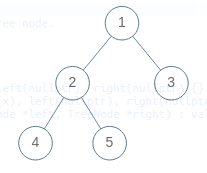Problem title
Difficulty
Avg time to solve

Move All Negative Numbers To Beginning And Positive To End
Easy
10 mins
Ninja Competition
Easy
--
Count Univalue Subtrees
Moderate
30 mins
Height of the Binary Tree From Inorder and Level Order Traversal
Moderate
10 mins
Delete Node In A Linked List
Easy
15 mins
Smallest Subarray With K Distinct Elements
Easy
20 mins
Maximum meetings
Easy
10 mins
Modular Exponentiation
Easy
15 mins
Maximum Distance
Moderate
15 mins
Convert String
Easy
15 mins46

# Height of the Binary Tree From Inorder and Level Order Traversal

Difficulty: MEDIUM
Avg. time to solve
10 min
Success Rate
90%

Problem Statement

#### The height of the binary tree is the number of edges in the longest path from the root node to any leaf node in the tree. In case the tree has only one node, the height is taken to be 0.

##### Input Format:
``````The first line of input contains an integer ‘T’ denoting the number of test cases to run. Then the test case follows.

The first line of each test case contains an integer  ‘N’ denoting the number of nodes of the binary tree.

The second line of each test case contains ‘N’ single space-separated integers, denoting the Inorder traversal of the binary tree.

The third line of each test case contains ‘N’ single space-separated integers, denoting the Level Order traversal of the binary tree.
``````
##### Output Format:
``````For each test case, print the height of the binary tree.

Output for every test case will be printed in a separate line.
``````
##### Note:
``````You do not need to print anything; it has already been taken care of. Just implement the given function.
``````
##### Constraints:
``````1 <= T <= 100
1 <= N <= 3000
1 <= inorder[i] <= N
1 <= levelOrder[i] <= N

Time Limit: 1 sec
``````
##### Sample Input 1:
``````1
5
4 2 5 1 3
1 2 3 4 5
``````
##### Sample Output 1:
``````2
``````
##### Explanation for Sample 1:
``````The binary tree(rooted at node 1) represented by the above inorder and level order traversals is-
````````````We can see that the height of the above binary tree is 2.
``````
##### Sample Input 2:
``````1
7
7 4 2 1 5 3 6
1 2 3 4 5 6 7
``````
##### Sample Output 2:
``````3
``````Console